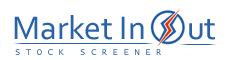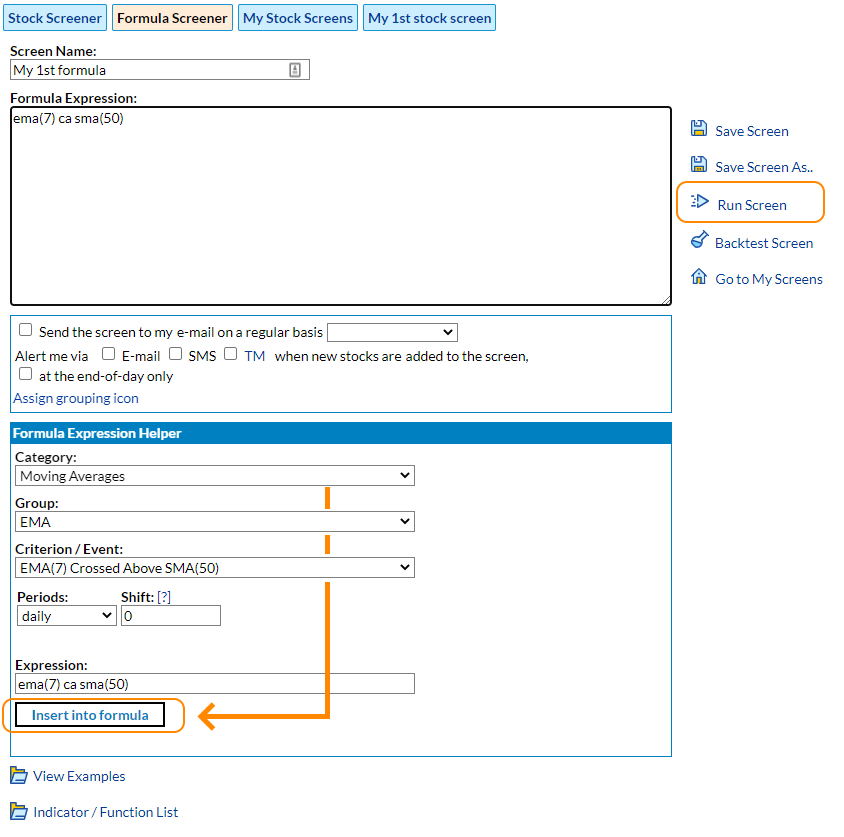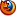Please enable JavaScript to view this page content properly Log In | Sign Up
Formula Screener

Formula Screener is the most powerful tool we provide in our service. Whereas regular stock screener applies "and" condition to all selected criteria (i.e. all criteria must be met for a stock to be selected), the Formula Screener allows you to combine "and", "or" and "not" conditions to build very specific criteria. Moreover, the Formula Screener tool gives you the possibility to combine criteria on different time periods. Other words, it is possible to find stocks that meet some conditions on a daily chart and other conditions on weekly or monthly charts. It worth to mention that the historical screening feature gets more flexible in the Formula Screener. You can specify offset value for any criteria in a formula expression in days or in weeks or months if the corresponding time periods were set for the criteria. Range screening leverages the above approach and provides you with the ability to set a condition to be met within some period of time. Let say you may allow moving average crossover to occur within last 3 days or require an oscillator to be in oversold zone all time last 10 days. Please note, that when combining many conditions in a formula, it is important to use parentheses so that the screener knows what order to evaluate each condition. The following examples demonstrate how to code maximally flexible criteria in formula expressions:

 Formula Example Explanation

 You may write a formula directly in the Formula text area or use Formula Expression Helper pane to build certain conditions and insert them into the formula one by one: Select criteria Category and Group first, then chose specific criterion or technical event you would like to add to the formula expression. The selected condition will appear in the Expression text field. It allows edits so you may adjust parameters before adding the condition to the formula. Hit Insert into formula button to add the condition to the formula expression. Add other conditions the same way if necessary and perform final edits of the expression in the Formula text area. To view matched stocks click on Run Screen link:The following table contains the full list of indicators that can be used in formula expression. They can also be accessed with the usage of Formula Expression Helper.
 Indicator List
 ac(5,34,5) Accelerator Oscillator ad Accumulation / Distribution advdec Advance/Decline Line advdecvol Advance/Decline Volume Line adx(14) Average Directional Movement Index ao(5,34) Awesome Oscillator aroon(25) Aroon Indicator aroonosc(25) Aroon Oscillator atr(14) Average True Range bs(5) Balance Step bb(20,2) Bollinger BandWidth, % bbands(20,2) Bollinger Bands bbub(20,2) Upper Band of Bollinger Bands bblb(20,2) Lower Band of Bollinger Bands beta(5) Beta bop Balance Of Power chmo(9) Chande Momentum Oscillator cho(3,10) Chaikin Oscillator chop(14) Choppiness Index cci(18) Commodity Channel Index cmf(20) Chaikin Money Flow crsi(3,2,100) Connors RSI dema(50) Double Exponential Moving Average dim(14) Negative Directional Indicator (-DI) dip(14) Positive Directional Indicator (+DI) ema(50) Exponential Moving Average ema(50,7) Forward shifted Exponential Moving Average. The extra "7" at the end is the shifting parameter. emah(50) Exponential Moving Average applied to high prices emal(50) Exponential Moving Average applied to low prices emae(50,20) Exponential Moving Average Envelope emaeub(50,20) Upper Bound of Exponential Moving Average Envelope emaelb(50,20) Lower Bound of Exponential Moving Average Envelope emarsi(14,9) Exponential Moving Average of RSI emv(14) Ease of Movement force(14) Elder Force Index hma(50) Hull Moving Average ichimoku(9,26,52) Ichimoku tenkan(9,26,52) Ichimoku, Tenkun Line kijun(9,26,52) Ichimoku, Kijun Line spana(9,26,52) Ichimoku, Span A Line spanb(9,26,52) Ichimoku, Span B Line chikou(9,26,52) Ichimoku, Chikou Line kama(30) Kaufman Adaptive Moving Average keltub(20,2,10) Keltner Upper Band keltlb(20,2,10) Keltner Lower Band macd(12,26,9) MACD macds(12,26,9) MACD Signal Line macdh(12,26,9) MACD Histogram mama(0.5,0.05) MESA Adaptive Moving Average mfi(14) Money Flow Index mhigh Monthly Previous High mlow Monthly Previous Low mclose Monthly Previous Close mi(25) Mass Index minusvi(14) Vortex Indicator -VI Line mo McClellan Oscillator mvo McClellan Volume Oscillator mom(12) Momentum msi McClellan Summation Index mvsi McClellan Volume Summation Index natr(14) Normalized Average True Range obv On Balance Volume pp Pivot Point ps1 Pivot Point (S1) ps2 Pivot Point (S2) pr1 Pivot Point (R1) pr2 Pivot Point (R2) plusvi(14) Vortex Indicator +VI Line ppo(12,26,9) Percentage Price Oscillator ppoh(12,26,9) PPO Histogram psar(0.02,0.2) Parabolic SAR range(5) Range roc(10) Price Rate of Change rci(10) Rank Correlation Index rmi(14,10) Relative Momentum Indicator rs5 1-Week Relative Strength rs10 2-Week Relative Strength rs21 1-Month Relative Strength rs68 3-Month Relative Strength rs126 6-Month Relative Strength rs252 1-Year Relative Strength rsi(14) Relative Strength Index sma(50) Simple Moving Average sma(50,7) Forward shifted Simple Moving Average. The extra "7" at the end is the shifting parameter. smah(50) Simple Moving Average applied to high prices smal(50) Simple Moving Average applied to low prices smae(50,20) Simple Moving Average Envelope smarsi(14,9) Simple Moving Average of RSI smi(5,3,3) Stochastic Momentum Indicator snr_res(9,1) The first resistance level of S&R indicator snr_sup(9,1) The first support level of S&R indicator snroc_res(9,1) The first resistance level of S&ROC indicator snroc_sup(9,1) The first support level of S&ROC indicator snrc_res(9,1) The first resistance level of S&RC indicator snrc_sup(9,1) The first support level of S&RC indicator stc(23,50,10) Schaff Trend Cycle stddev(5,1) Standard Deviation stoch(5,3,3) Stochastic Oscillator (fast %K, slow %K, slow %D) slowk(5,3,3) Stochastic Oscillator (fast %K, slow %K, slow %D), slow %K Line slowd(5,3,3) Stochastic Oscillator (fast %K, slow %K, slow %D), slow %D Line stochrsi(14) Stochastic RSI supertrend(3,7) SuperTrend Indicator tema(50) Triple Exponential Moving Average tmf(21) Twiggs Money Flow trix(14,8) Triple Exponential Average trixs(14,8) Triple Exponential Average Signal Line tsi(25,13) True Strength Index uo(7,14,28) Ultimate Oscillator udon(20) Upper Donchian mdon(20) Middle Donchian ldon(20) Lower Donchian ulr(100,2) Upper Line of Linear Regression Channel (period, number of standard deviations) mlr(100,2) Middle Line of Linear Regression Channel (period, number of standard deviations) llr(100,2) Lower Line of Linear Regression Channel (period, number of standard deviations) vma(20) Volume Moving Average vo(7,14) Volume Oscillator vr(21) Volume Ratio vwma(50) Volume Weighted Moving Average wad William's Accumulation / Distribution wavetrend(10,21) WaveTrend Oscillator wts(10,21) WaveTrend Signal Line wild(21) Wilder Moving Average wma(50) Weighted Moving Average wma(50,7) Forward shifted Weighted Moving Average. The extra "7" at the end is the shifting parameter. wpr(14) Williams` Percent Range whigh Weekly Previous High wlow Weekly Previous Low wclose Weekly Previous Close yhigh Yealy Previous High ylow Yealy Previous Low yclose Yealy Previous Close zigzag(7) ZigZag

 Technical Events
 Event Explanation Formula sample ca Crossed Above ema(7) ca ema(50) cb Crossed Below ema(7) cb ema(50) ia Is Above price ia ema(50) ib Is Below price ib ema(50) tocha Touched Above price tocha sma(50) tochb Touched Below price tochb sma(50) bon_up Bounced Up From price bon_up bblb(20,2) bon_dn Bounced Down From price bon_dn bbub(20,2) div_bull Bullish Divergence rsi(14) div_bull div_bear Bearish Divergence rsi(14) div_bear trend_up Trending Up price trend_up 7 trend_dn Trending Down price trend_dn 7

 Function Example Explanation
 Highest and lowest prices highest(10) Returns the highest price over a specific number of days/bars lowest(10) Returns the lowest price over a specific number of days/bars highestbar(10) Returns the shift of the highest price over a specific number of days/bars lowestbar(10) Returns the shift of the lowest price over a specific number of days/bars highestvol(10) Returns the highest volume value over a specific number of days/bars lowestvol(10) Returns the lowest volume value over a specific number of days/bars highestvolbar(10) Returns the shift of the highest volume value over a specific number of days/bars lowestvolbar(10) Returns the shift of the lowest volume value over a specific number of days/bars highestclose(10) Returns the highest close price over a specific number of days/bars lowestclose(10) Returns the lowest close price over a specific number of days/bars highestoc(10) Returns the highest open or close price over a specific number of days/bars lowestoc(10) Returns the lowest open or close price over a specific number of days/bars highestclosebar(10) Returns the shift of the highest close price over a specific number of days/bars lowestclosebar(10) Returns the shift of the lowest close price over a specific number of days/bars highestocbar(10) Returns the shift of the highest open or close price over a specific number of days/bars lowestocbar(10) Returns the shift of the lowest open or close price over a specific number of days/bars Stock universe ticker(fb,aapl,msft) The ticker function allows you to run the screener only on the ticker names specified as parameters. May not include more than 100 ticker names. Use the Watch List tool to run the screener on a larger ticker list. Calendar functions day(1) Returns true if current day of month equals to the parameter value. The parameter value must be in range of 1..31 weekday(Sun) Returns true if current day of week equals to the parameter value. The parameter value must be in range of Sun..Sat month(Jan) Returns true if current month equals to the parameter value. The parameter value must be in range of Jan..Dec Candlestick functions wick(0) Returns the size of the "upper shadow" part of a candle. The parameter value is the shift relative to the current bar tail(0) Returns the size of the "lower shadow" part of a candle. The parameter value is the shift relative to the current bar body(0) Returns the size of the "real body" part of a candle. The parameter value is the shift relative to the current bar crange(0) The range the price moved during the time frame of the candlestick (high - low). The parameter value is the shift relative to the current bar bmid(0) The middle of the candlestick's "real body" (close + open) / 2. The parameter value is the shift relative to the current bar tprice(0) Returns the Typical Price value for a given bar: (high + low + close) / 3. The parameter value is the shift relative to the current bar mprice(0) Returns the Median Price value for a given bar: (high + low) / 2. The parameter value is the shift relative to the current bar wprice(0) Returns the Weighted Price value for a given bar: (high + low + 2 * close) / 4. The parameter value is the shift relative to the current bar Price level functions fibo23s(1) Returns the value of the 23.6% Fibonacci retracement level. Fibonacci is applied to the first (nearest by registering date) recognized uptrend. Examples: price cb fibo23s(1) - price crossed below the 23.6% fibo level. price tocha fibo23s(1) - price touched above the 23.6% fibo level. price bon_up fibo23s(1) - price bounced up from the 23.6% fibo level. For 38.2%, 50.0%, 61.8% and 78.6% fibonacci levels, you can use the following functions: fibo38s, fibo50s, fibo62s, fibo78s. fibo23r(1) Returns the value of the 23.6% Fibonacci retracement level. Fibonacci is applied to the first (nearest by registering date) recognized downtrend. Examples: price ca fibo23r(1) - price crossed above the 23.6% fibo level. price tochb fibo23r(1) - price touched below the 23.6% fibo level. price bon_dn fibo23r(1) - price bounced down from the 23.6% fibo level. For 38.2%, 50.0%, 61.8% and 78.6% fibonacci levels, you can use the following functions: fibo38r, fibo50r, fibo62r, fibo78r. snr_sup(9,1) Returns the value of the first (nearest by registering date) support level of the SNR(9) indicator. Examples: price cb snr_sup(9,1) - price crossed below the 1st support level of the SNR(9) indicator. price tocha snr_sup(9,2) - price touched above the 2nd support level of the SNR(9) indicator. price bon_up snr_sup(9,3) - price bounced up from the 3nd support level of the SNR(9) indicator. snr_res(9,1) Returns the value of the first (nearest by registering date) resistance level of the SNR(9) indicator. Examples: price ca snr_res(9,1) - price crossed above the 1st resistance level of the SNR(9) indicator. price tochb snr_res(9,2) - price touched below the 2nd resistance level of the SNR(9) indicator. price bon_dn snr_res(9,3) - price bounced down from the 3nd resistance level of the SNR(9) indicator. Aggregate functions abs(rsi(14) - 50) Returns the absolute (positive) value of an expression max(rsi(14), 10) Returns the maximum value of an expression for the specified number of days / bars min(rsi(14), 10) Returns the minimum value of an expression for the specified number of days / bars avg(rsi(14), 10) Returns the average value of an expression for the specified number of days / bars count(rsi(14) < 30, 10) Returns the number of days / bars for which the expression is true for the specified number of days / bars change(10) Returns the price % change over the specified number of days / bars evalat(sma(21) - sma(7), 10) Returns the value of the expression specified as the first parameter at the day/bar specified in the second parameter. The expression in this example works the same way as historical screening "(sma(21) - sma(7))@10" but the second parameter can contain any arithmetic expression, for example: evalat(sma(21) - sma(7), min(rsi(14), 7)) Math functions math.abs(rsi(14) - 50) Returns the absolute (positive) value of an expression math.max(ema(50), ema(200)) Returns the largest of two values passed to it as parameter math.min(ema(50), ema(200)) Returns the smallest of two values passed to it as parameter math.sqrt(rsi(14)) Calculates the square root of the parameter given to it math.pow(rsi(14), 2) The method returns the value of the first parameter raised to the power of the second parameter math.round(rsi(14)) Rounds a floating point value to the nearest integer using normal math round rules (either up or down) math.ceil(rsi(14)) Rounds a floating point value up to the nearest integer value math.floor(rsi(14)) Rounds a floating point value down to the nearest integer value Backtesting aids posprice Formula expression can be used as a criteria for closing a position in the Strategy Backtest tool. This can be done by ticking the "Set by a formula expression" option and assigning the corresponding formula expression. You can get the value of the price at which the position was opened using the "posprice" literal. posbar You can get the index of the day/bar at which the position was opened using the "posbar" literal. It is typically used in data arrays: open, high, low, close, v. For example, low[posbar] will give the low price of the day/bar at which the position was opened. evalat You can get the value of the indicator or expression at the moment the position was opened using the "posbar" literal and the "evalat" function. For example, evalat(rsi(14), posbar) will give the value of the RSI(14) indicator recorded on the day the position was opened. posage You can get the number of days/bars elapsed since the position was opened. Time functions last_update() Returns the number of minutes since the last stock quote update

 Content Overview  Data  Stock Screener       Quick Start       Screener Parameters       How to Screen Watch List       Formula Screener       Technical Criteria  Strategy Backtest       New Strategy       How It Works       Testing Model       Position Maintenance       How to Backtest Watch List  Charts  Portfolio Tracker  Trade Alert  Price Alert  Stock Correlation

 Best viewed in